SLVSCQ4 October   2014

PRODUCTION DATA.

1. Features
2. Applications
3. Description
4. Typical Application Circuit (Multiple Daisy Chained TLC5957s)
5. Revision History
6. Pin Configuration and Functions
7. Specifications
8. Parameter Measurement Information
9. Detailed Description
1. 9.1 Overview
2. 9.2 Functional Block Diagram
3. 9.3 Device Functional Modes
10. 10Application and Implementation
11. 11Power Supply Recommendations
12. 12Layout
13. 13Device and Documentation Support
14. 14Mechanical, Packaging, and Orderable Information

• RTQ|56
• RTQ|56

## 7 Specifications

### 7.1 Absolute Maximum Ratings

over operating free-air temperature range (unless otherwise noted) (1)
MIN MAX UNIT
VCC(2) Supply voltage VCC –0.3 6.0 V
IOUT Output current (dc) OUTx0 to OUTx15, x = R, G, B 30 30 mA
VIN(2) Input voltage range SIN, SCLK, LAT, GCLK, IREF –0.3 VCC + 0.3 V
VOUT(2) Output voltage range SOUT –0.3 VCC + 0.3 V
OUTx0 to OUTx15, x = R, G, B –0.3 11 V
TJ(MAX) Operation junction temperature –40 150 °C
(1) Stresses beyond those listed under Absolute Maximum Ratings may cause permanent damage to the device. These are stress ratings only, which do not imply functional operation of the device at these or any other conditions beyond those indicated under Recommended Operating Conditions. Exposure to absolute-maximum-rated conditions for extended periods may affect device reliability.
(2) All voltage values are with respect to device ground terminal.

### 7.2 Handling Ratings

MIN MAX UNIT
Tstg Storage temperature range –55 150 °C
V(ESD) Electrostatic discharge Human body model (HBM), per ANSI/ESDA/JEDEC JS-001, all pins(1) –3 3 kV
Charged device model (CDM), per JEDEC specification JESD22-C101, all pins(2) –1 1
(1) JEDEC document JEP155 states that 500-V HBM allows safe manufacturing with a standard ESD control process.
(2) JEDEC document JEP157 states that 250-V CDM allows safe manufacturing with a standard ESD control process.

### 7.3 Recommended Operating Conditions

At TA = –40°C to 85°C, unless otherwise noted.
MIN NOM MAX UNIT
DC CHARACTERISTICS VCC = 3 V to 5.5 V
VCC Supply voltage 3 5.5 V
VO Voltage applied to output OUTx0 to OUTx15, x = R, G, B 10 V
VIH High level input voltage SIN, SCLK, LAT, GCLK 0.7×VCC VCC V
VIL Low level input voltage SIN, SCLK, LAT, GCLK GND 0.3×VCC V
IOH High level output current SOUT –2 mA
IOL Low level output current SOUT 2 mA
IOLC Constant output sink current OUTx0 to OUTx15, x = R, G, B, 3V ≤ VCC ≤ 4V 20 mA
OUTx0 to OUTx15, x = R, G, B, 4V < VCC ≤ 5.5V 25
TA Operating free air temperature –40 85 °C
TJ Operation junction temperature –40 125 °C
AC CHARACTERISTICS, VCC = 3 V to 5.5 V
FCLK(SCLK) Data shift clock frequency SCLK 33 MHz
FCLK(GCLK) Grayscale control clock frequency GCLK 33 MHz

### 7.4 Thermal Information

THERMAL METRIC(1) TLC5957 UNIT
RTQ (56 PINS)
RθJA Junction-to-ambient thermal resistance 27.4 °C/W
RθJC(top) Junction-to-case (top) thermal resistance 13.6
RθJB Junction-to-board thermal resistance 5.5
ψJT Junction-to-top characterization parameter 0.2
ψJB Junction-to-board characterization parameter 5.5
RθJC(bot) Junction-to-case (bottom) thermal resistance 0.8

### 7.5 Electrical Characteristics

over operating free-air temperature range (unless otherwise noted)
PARAMETER TEST CONDITIONS MIN TYP MAX UNIT
VOH Output voltage High IOH = –2mA at SOUT VCC-0.4 VCC V
VOL Low IOL = 2mA at SOUT 0.4 V
VLOD0 LED open detection threshold LODVTH = 00b 0.05 0.09 0.15 V
VLOD1 LODVTH = 01b 0.15 0.19 0.25 V
VLOD2 LODVTH = 10b 0.3 0.35 0.4 V
VLOD3 LODVTH = 11b 0.45 0.49 0.55 V
VIREF Reference voltage output RIREF = 5.97kΩ　(1mA target), BC = 0h, CCR/G/B = 80h 1.184 1.209 1.234 V
IIN Input current (SIN, SCLK, LAT, GCLK) VIN = VCC or GND –1 1 µA
ICC0 Supply current (VCC) SIN/SCLK/LAT/GSCLK = GND, GSn = 0000h, BC = 4h, CCR/G/B = 120h,
VOUTn = 0.6V, RIREF = OPEN, VCC = 4V
8 10 mA
ICC1 SIN/SCLK/LAT/GSCK = GND, GSn = 0000h, BC = 4h, CCR/G/B = 120h,
VOUTn = 0.6V, RIREF = 7.5kΩ (Io = 10mA target) , VCC = 4V
11 13
ICC2 SIN/SCLK/LAT = GND, GCLK = 33MHz, TSU3 = 200ns, XREFRESH = 0,
GSn = FFFFh, BC = 4h, CCR/G/B = 120h, VOUTn = 0.6V,
RIREF = 7.5kΩ (Io = 10mA target) , VCC = 4V
20 26
ICC3 SIN/SCLK/LAT = GND, GCLK = 33MHz, TSU3 = 200ns, XREFRESH = 0,
GSn = FFFFh, BC = 7h, CCR/G/B = 1D2h, VOUTn = 0.6V,
RIREF = 7.5kΩ (Io = 25mA target) , VCC = 4V
22 28
ICC4 In power save mode 0.9 1.5
Δ IOLC0 Constant current error (OUTx0-15, x = R/G/B) Channel-to-channel(1) All OUTn = on, BC = 0h, CCR/G/B = 08Fh, VOUTn = VOUTfix = 0.6V,
RIREF = 7.5kΩ(1mA target), TA = +25C, at same color grouped output of
OUTR0-15, OUTG0-15 and OUTB0-15
±1% ±3%
ΔIOLC1 Constant current error (OUTx0-15, x = R/G/B) Device-to-device(2) All OUTn = on, BC = 0h, CCR/G/B = 08Fh, VOUTn = VOUTfix = 0.6V,
RIREF = 7.5kΩ(1mA target), TA = +25C, at same color grouped output of
OUTR0-15, OUTG0-15 and OUTB0-15
±1% ±2%
Δ IOLC2 Line regulation(3) VCC = 3.0 to 5.5V, All OUTn = on, BC = 0h, CCR/G/B = 08Fh,
VOUTn = VOUTfix = 0.6V, RIREF = 7.5kΩ　(1mA target)
±1 ±3 %/V
ΔIOLC3 Load regulation(4) VCC = 4V, All OUTn = on, BC = 0h, CCR/G/B = 08Fh, VOUTn = 0.6 to 3V,
VOUTfix = 1V, RIREF = 7.5kΩ　(1mA target)
±1 ±3 %/V
ΔIOLC4 Constant current error (OUTx0-15, x = R/G/B) Channel-to-channel(1) All OUTn = on, BC = 7h, CCR/G/B - 1CCh, VOUTn = VOUTfix = 0.6V,
RIREF = 7.5kΩ(25mA target), TA = +25C, at same color grouped output of OUTR0-15, OUTG0-15 and OUTB0-15
±1% ±3%
ΔIOLC5 Constant current error (OUTx0-15, x = R/G/B) Device-to-device(2) All OUTn = on, BC = 7h, CCR/G/B - 1CCh, VOUTn = VOUTfix = 0.6V,
RIREF = 7.5kΩ(25mA target), TA = +25C, at same color grouped output of OUTR0-15, OUTG0-15 and OUTB0-15
±1% ±3%
ΔIOLC6 Line regulation(3) VCC = 3.0 to 5.5V, All OUTn = on, BC = 7h, CCR/G/B - 1CCh,
VOUTn = VOUTfix = 0.6V, RIREF = 7.5kΩ　(25mA target)
±1 ±3 %/V
Δ IOLC7 Load regulation(4) All OUTn = on, BC = 7h, CCR/G/B - 1CCh, VOUTn = 0.6 to 3V,
VOUTfix = 0.6V, RIREF = 7.5kΩ　(25mA target)
±1 ±3 %/V
TTSD Thermal shutdown threshold 160 170 180 °C
THYS Thermal shutdown hysterisis 10 °C
VISP(in) IREF resistor short protection threshold 0.190 V
VISP(out) IREF resistor short-protection release threshold 0.330 V
(1) The deviation of each outputs in same color group (OUTR0~15 or OUTG0~15 or OUTB0~15) from the average of same color group constant current. The deviation is calculated by the formula. (X = R or G or B, n = 0~15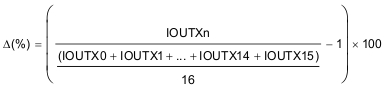(2) The deviation of the average of constant-current in each color group from the ideal constant-current value. (X = R or G or B) :Ideal current is calculated by the following equation: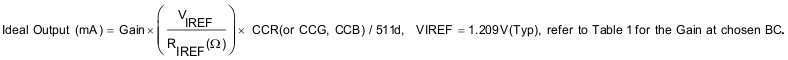(3) Line regulation is calculated by the following equation. (X = R or G or B, n = 0~15):(4) Load regulation is calculated by the following equation. (X = R or G or B, n = 0~15):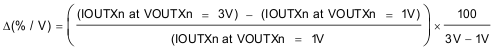### 7.6 Timing Requirements

At TA = –40°C to 85°C, unless otherwise noted.
MIN TYP MAX UNIT
AC CHARACTERISTICS, VCC = 3 V to 5.5 V
tWH0 Pulse duration SCLK 10 ns
tWL0 SCLK 10 ns
tWH1 GCLK 10 ns
tWL1 GCLK 10 ns
tWH2 LAT 10 ns
tSU0 Setup time SIN – SCLK↑ 2 ns
tSU1 LAT↑ – SCLK↑ 3 ns
tSU2 LAT↓ – SCLK↑ , for WRTGS, WRTFC, and TMGST Command 20 ns
LAT↓ – SCLK↑ , for LATGS, READFC, and LINERESET Command 80 ns
tSU3 LAT↓ – GCLK↑ , for LATGS AND LINERESET Command 30 ns
tH0 SCLK↑ – SIN 2 ns
tH1 SCLK↑ – LAT↑ 2 ns
tH2 SCLK↓ – LAT↓ 2 ns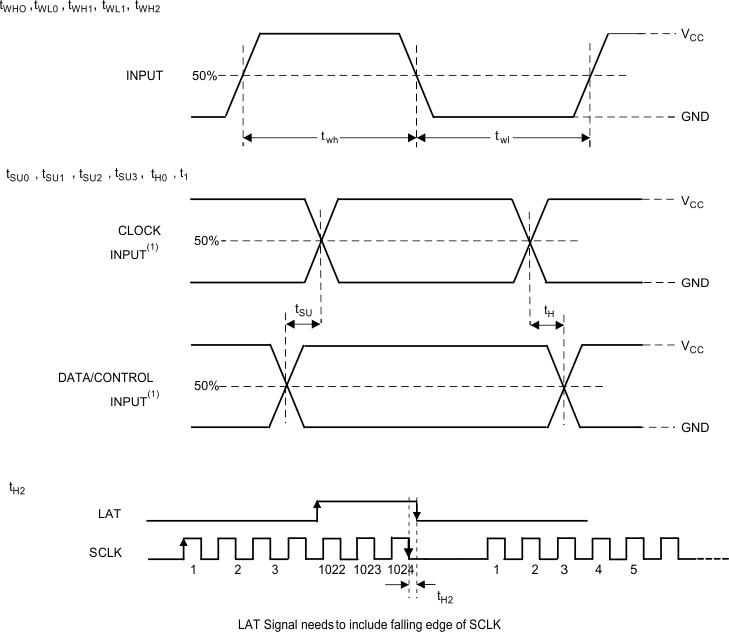Figure 1. Input Timing

### 7.7 Typical Characteristics

At VCC= 4V and TA = 25°C, unless otherwise noted.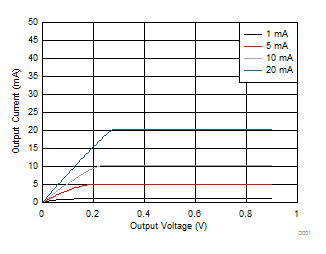VCC = 4 V
Figure 2. Output Current vs Output Voltage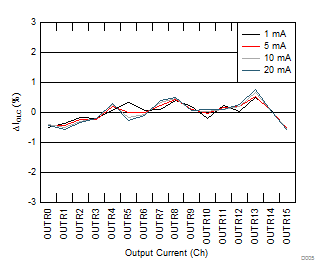VCC = 4 V
Figure 4. Constant-Current Error vs Output Current
(Channel-to-Channel in RED color group)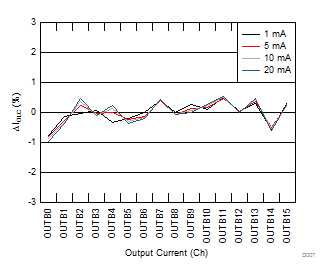VCC = 4 V
Figure 6. Constant-Current Error vs Output Current
(Channel-to-Channel in BLUE color group)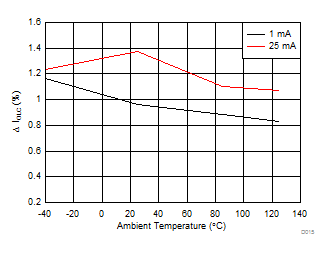VCC=4V VOUTXn=0.6V
Figure 8. Maximum Constant-Current Error vs Ambient Temperature
(Channel-to-Channel in GREEN color group)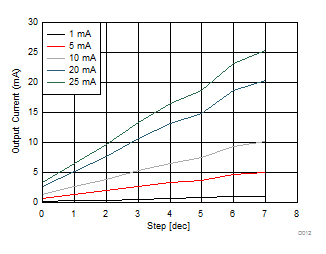Figure 10. Global Brightness Control Linearity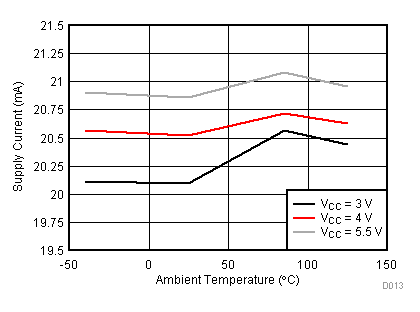GCLK=33MHz VOUTXn=0.6V, Output Current=10mA, GSXn=FFFFh,
Figure 12. Supply Current vs Ambient Temperature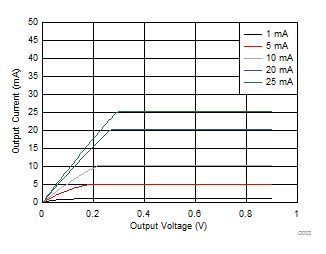VCC = 5 V
Figure 3. Output Current vs Output Voltage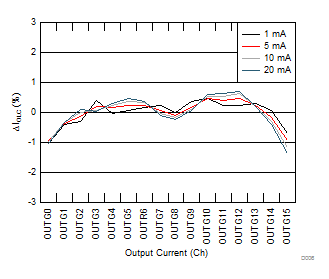VCC = 4 V
Figure 5. Constant-Current Error vs Output Current
(Channel-to-Channel in GREEN color group)VCC=4V VOUTXn=0.6V
Figure 7. Maximum Constant-Current Error vs Ambient Temperature
(Channel-to-Channel in RED color group)VCC=4V VOUTXn=0.6V
Figure 9. Maximum Constant-Current Error vs Ambient Temperature
(Channel-to-Channel in BLUE color group)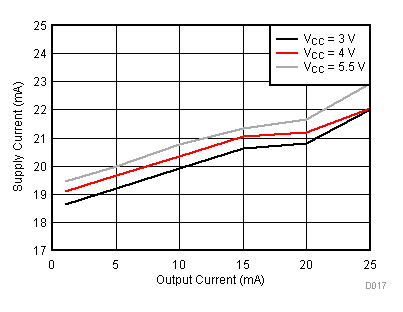VOUTXn=0.6V, GCLK=33MHz, GSXn=FFFFh,
Figure 11. Supply Current (ICC) vs Output Current